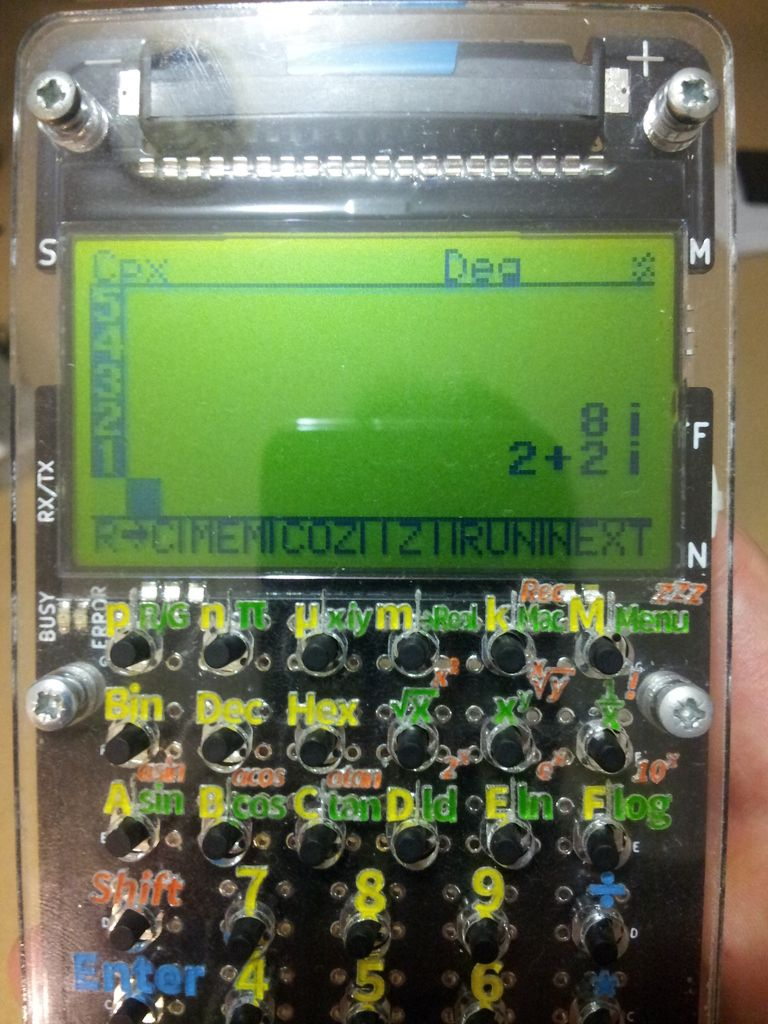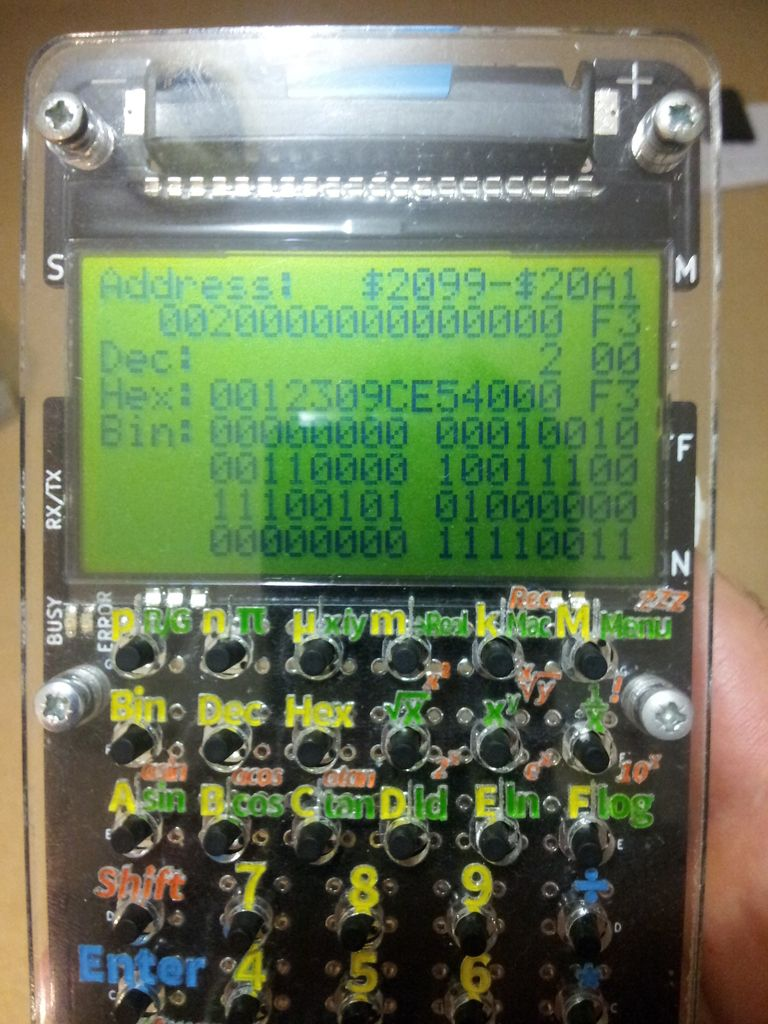(Free42) roundoff for complex SQRT - Printable Version +- HP Forums (https://www.hpmuseum.org/forum) +-- Forum: Not HP Calculators (/forum-7.html) +--- Forum: Not quite HP Calculators - but related (/forum-8.html) +--- Thread: (Free42) roundoff for complex SQRT (/thread-10440.html) Pages: 1 2 (Free42) roundoff for complex SQRT - Werner - 04-03-2018 01:28 PM Nitpicking, perhaps, but if the original 42S gets it right - then so should Free42 ;-) Try SQRT(a+ib) with a=0 and b/2 a perfect square eg. b=8 Free42 shows 2+i2, which SHOW reveals to be really 2+i(2-1E-33). Almost any b you throw at it has a rounding error like that, in the real or complex part. The 12-digit 42S gets the exact result - it must have special code for a=0, and return SQRT(ABS(b)/2)*(1+i*SIGN(b)) in that case - even when b/2 would underflow. Cheers, Werner RE: (Free42) roundoff for complex SQRT - Claudio L. - 04-03-2018 01:44 PM (04-03-2018 01:28 PM)Werner Wrote:  The 12-digit 42S gets the exact result - it must have special code for a=0, and return SQRT(ABS(b)/2)*(1+i*SIGN(b)) in that case - even when b/2 would underflow. It must have some kind of special code. I had the same artifacts and had to add special code in newRPL to detect tiny values in the imaginary part. If found, then the real part is tried by itself as a root, and if it satisfies the equation the real root is returned instead (sort of "snap" to the real axis). RE: (Free42) roundoff for complex SQRT - Thomas Okken - 04-03-2018 01:48 PM Yup, there's no special case for pure imaginary in SQRT. It looks like this fell between the cracks when I did the grand cleanup of pure-real and pure-imaginary complex transcendentals last year. Because SQRT is not a transcendental function, I guess.RE: (Free42) roundoff for complex SQRT - zeno333 - 04-03-2018 05:29 PM Will this be corrected in Free42, and will that correction also make it to DM42 Firmware or are the days of the DM42 updates to the latest Free42 over now??? RE: (Free42) roundoff for complex SQRT - Thomas Okken - 04-03-2018 05:43 PM (04-03-2018 05:29 PM)zeno333 Wrote:  Will this be corrected in Free42, and will that correction also make it to DM42 Firmware or are the days of the DM42 updates to the latest Free42 over now??? 1. Sure!!! 2. They download the updates from my web site!!! Even if they continue to flout the GPL, I can't stop them from doing that!!! Although I would like to!!! Why are you using so many question marks??? RE: (Free42) roundoff for complex SQRT - Werner - 04-04-2018 07:11 AM This is the algorithm for complex square root as used in the 48G and up - it's probably the same as in the 42S: Code: ```*   Let R = SQRT(X^2+Y^2), A = SQRT((R+ABS(X))/2), B = Y/(2*A) *       Then *                     { (0,0)                   (X,Y) = (0,0) *       SQRT((X,Y)) = { (A,B)                   X >= 0 *                     { (ABS(B), SIGN(B)*A)     X < 0``` So no exception needs to be made for X=0 to get exact results for Y/2 being a perfect square. BTW the 42S is bound to exhibit less roundoff errors (meaning, exact results where possible) as all functions are computed using higher internal precision - what Free42 does not do. But in this case, it can be avoided ;-) Cheers, Werner RE: (Free42) roundoff for complex SQRT - Thomas Okken - 04-04-2018 07:25 AM If I'm going to check for Re(X)=0, cases where Im(X)/2 is a perfect square should return exact results, or at least no worse than SQRT for real arguments. That 48G code doesn't check for pure-real or pure-imaginary arguments, but Free42 uses such checks in lots of places, for various reasons, so it doesn't bother me to add one to complex SQRT as well.RE: (Free42) roundoff for complex SQRT - Dan - 04-04-2018 10:24 AM I used the "Friden" algorithm for square root on the AriCalculator, and sqrt(z) = sqrt(.5(a+sqrt(a^2+b^2)) )+ sqrt(0.5(-a+sqrt(a^2+b^2))). This gives exact results for 8i and other z I tried for which Re(z) = 0 and Im(z)/2 is a perfect square:The second image shows the value of the real and imaginary parts of the square root in memory (2nd line from top). I know nothing about the 42S, but I know early HP calculators used the "Friden" algorithm for square root. Is it possible the 42S did too? RE: (Free42) roundoff for complex SQRT - Claudio L. - 04-04-2018 03:03 PM (04-04-2018 10:24 AM)Dan Wrote:  I used the "Friden" algorithm for square root on the AriCalculator, and sqrt(z) = sqrt(.5(a+sqrt(a^2+b^2)) )+ sqrt(0.5(-a+sqrt(a^2+b^2))). This gives exact results for 8i and other z I tried for which Re(z) = 0 and Im(z)/2 is a perfect square This is what I used on newRPL too, but I'm probably going to change it to what Werner showed above for the 48, it saves one square root, so it's roughly 30% faster. RE: (Free42) roundoff for complex SQRT - Dan - 04-05-2018 09:36 AM That's really cool, thanks Claudio. I'll use the microcontroller's timer to compare performance and report the results. RE: (Free42) roundoff for complex SQRT - Dieter - 04-09-2018 08:22 PM (04-03-2018 01:28 PM)Werner Wrote:  Free42 shows 2+i2, which SHOW reveals to be really 2+i(2-1E-33). Almost any b you throw at it has a rounding error like that, in the real or complex part. The 12-digit 42S gets the exact result - it must have special code for a=0, and return SQRT(ABS(b)/2)*(1+i*SIGN(b)) in that case - even when b/2 would underflow. I assume (!) that the reason why a physical 42s returns an exact result simply is its three guard digits: the function is calculated to 15 digits, and if this yields 2,00000000000012 + 1,99999999999996 i this does not become visible as it is rounded to 12 digits before it is returned to the user. I wonder if Free42 (or DM42) make use of such guard digits. Is it possible that here these calculations are performed with the same 34 digits that are finally returned? This would explain slight errors in the last digit. Or are there really some additional digits that would cover roundoff errors like the ones discussed here? Edit: I just noticed that the use of guard digits has already been mentioned in your later post. Dieter RE: (Free42) roundoff for complex SQRT - Werner - 04-10-2018 07:14 AM Sadly, the Intel decimal floating-point library does not provide an extended format beyond 34 digits (double), so in Free42 the last digit of transcendental functions may be off, complex operations may exhibit (more) roundoff and there is no gain to be had in matrix dot products. Werner RE: (Free42) roundoff for complex SQRT - Paul Dale - 04-10-2018 08:11 AM If there is a fused multiply add call in Intel's decimal library, then dot products can be made accurate. It is computationally expensive to do so but it's not too difficult. Pauli RE: (Free42) roundoff for complex SQRT - Thomas Okken - 04-10-2018 05:28 PM (04-10-2018 08:11 AM)Paul Dale Wrote:  If there is a fused multiply add call in Intel's decimal library, then dot products can be made accurate. It is computationally expensive to do so but it's not too difficult. How would that work? (I'm not saying I'll do it, but I am curious. And the Intel library does have FMA.) RE: (Free42) roundoff for complex SQRT - Paul Dale - 04-10-2018 10:38 PM I can't find the reference I was thinking ofI'll keep looking. Pauli RE: (Free42) roundoff for complex SQRT - BarryMead - 04-11-2018 12:26 AM (04-10-2018 05:28 PM)Thomas Okken Wrote:   (04-10-2018 08:11 AM)Paul Dale Wrote:  If there is a fused multiply add call in Intel's decimal library, then dot products can be made accurate. It is computationally expensive to do so but it's not too difficult. How would that work? (I'm not saying I'll do it, but I am curious. And the Intel library does have FMA.) I found an article that shows how to use fma to do dot product and explains why it is more accurate. The Intel Decimal 128 bit FMA has several internal calculations performed at 256 bit accuracy, this is how it can be more accurate than separate multiply and add operations. https://www.quora.com/Floating-Point-How-does-Fused-Multiply-Add-FMA-work-and-what-is-its-importance-in-computing Note, however, that the order of the parameters in this example are backwards from those of the Intel Decimal library's FMA: In this article the order is Parameter1 + (Parameter2 * Parameter3), whereas in the Intel Decimal FMA the order is (Parameter1 * Parameter2) + Parameter3. I don't know if this is helpful or even applicable to your Complex Square Root algorithm, but I thought you might find it worth a look. RE: (Free42) roundoff for complex SQRT - Thomas Okken - 04-11-2018 01:09 AM Thanks! And no, this has nothing to do with complex SQRT, other than that both are operations whose accuracy could be improved. This is all going on my list of things to look at during the next burst of activity, eventually leading to release 2.0.21. RE: (Free42) roundoff for complex SQRT - Paul Dale - 04-11-2018 01:32 AM I hate it when I can't find a reference. The idea is to build a correctly rounded cross product from which a two dimensional dot product and complex multiplication can be done. I seem to remember it taking three FMA operations instead of two multiplications. FMA can also be useful for statistical accumulations and polynomial evaluations using Horner's method. I'm sure I'll remember eventually, Pauli RE: (Free42) roundoff for complex SQRT - Werner - 04-11-2018 09:02 AM Found this (see attachment). Apparently performing the dot product as before, and carrying along a correction term that can be determined with the FMA (a bit like Kahan summation for sums), results in a dot product as accurate as if it were computed in double precision. Remark that the FMA is only used to determine the correction factor - when FMA's are used for the dot product itself it is much more costly. Also, in this case, the computational penalty is 63% for n=50, and apparently going down to 25% for large n. Keyword to google is 'compensated dot product FMA' Cheers, Werner RE: (Free42) roundoff for complex SQRT - Dan - 05-11-2018 09:06 AM (04-04-2018 03:03 PM)Claudio L. Wrote:   (04-04-2018 10:24 AM)Dan Wrote:  I used the "Friden" algorithm for square root on the AriCalculator, and sqrt(z) = sqrt(.5(a+sqrt(a^2+b^2)) )+ sqrt(0.5(-a+sqrt(a^2+b^2))). This gives exact results for 8i and other z I tried for which Re(z) = 0 and Im(z)/2 is a perfect square This is what I used on newRPL too, but I'm probably going to change it to what Werner showed above for the 48, it saves one square root, so it's roughly 30% faster. Actually on my system it's slower (2 - 3 times), probably because I'm using a shift and add algorithm for division, so division is much slower than square root.